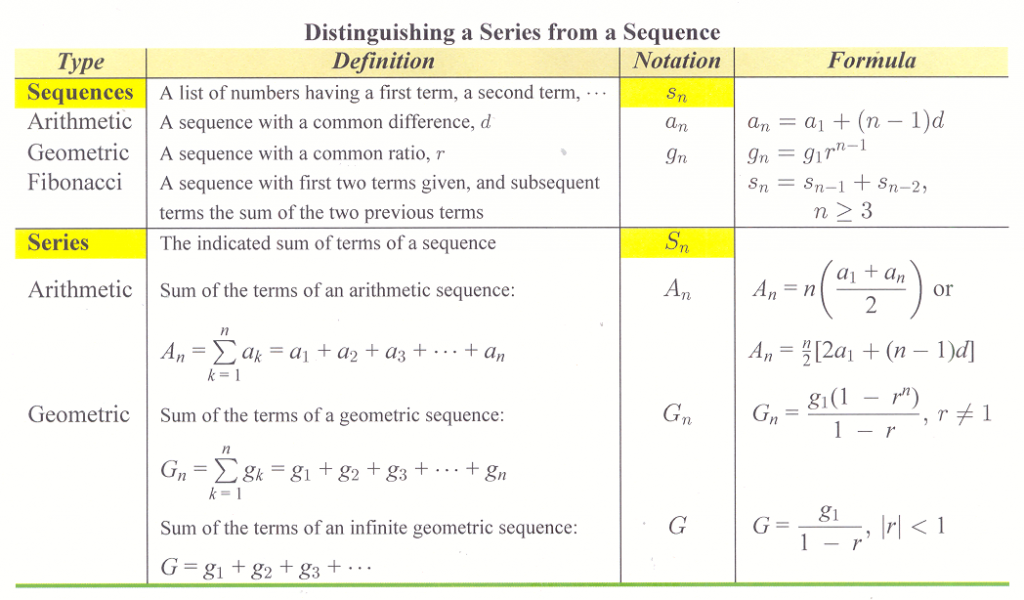# Section 11.4: Series

## 11.4 Outline

1. Finite series
1. define series
2. definition
3. alternating series
2. Summation notation
1. definition
2. sigma notation
3. evaluate a summation
4. expand a summation
3. Arithmetic series
1. definition
2. formula
4. Geometric series
1. definition
2. formula
5. Infinite geometric series
1. definition
2. partial sums
3. formula
6. Summary of sequence and series formulas

## 11.4 Essential Ideas

If the terms of sequence are added, the expression is called a series.

The indicated sum of the terms of a finite sequence s1, s2, s3, …, sis called a finite series and is denoted by  Sn s1+ s2+s3++sn.

An arithmetic series is the sum of the terms of an arithmetic sequence.

A geometric series is the sum of the terms of a geometric sequence.

If g1, g2, g3, …, g n, … is an infinite geometric sequence with a common ratio r such that |r| < 1, then the sum is denoted by G and is found by G = g1/(1 – r).  If |r| is greater than or equal to 1, then the infinite geometric series has no sum.